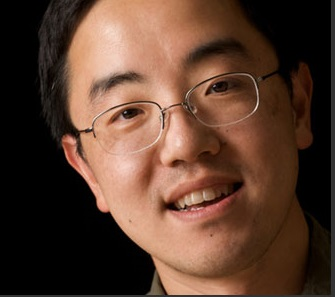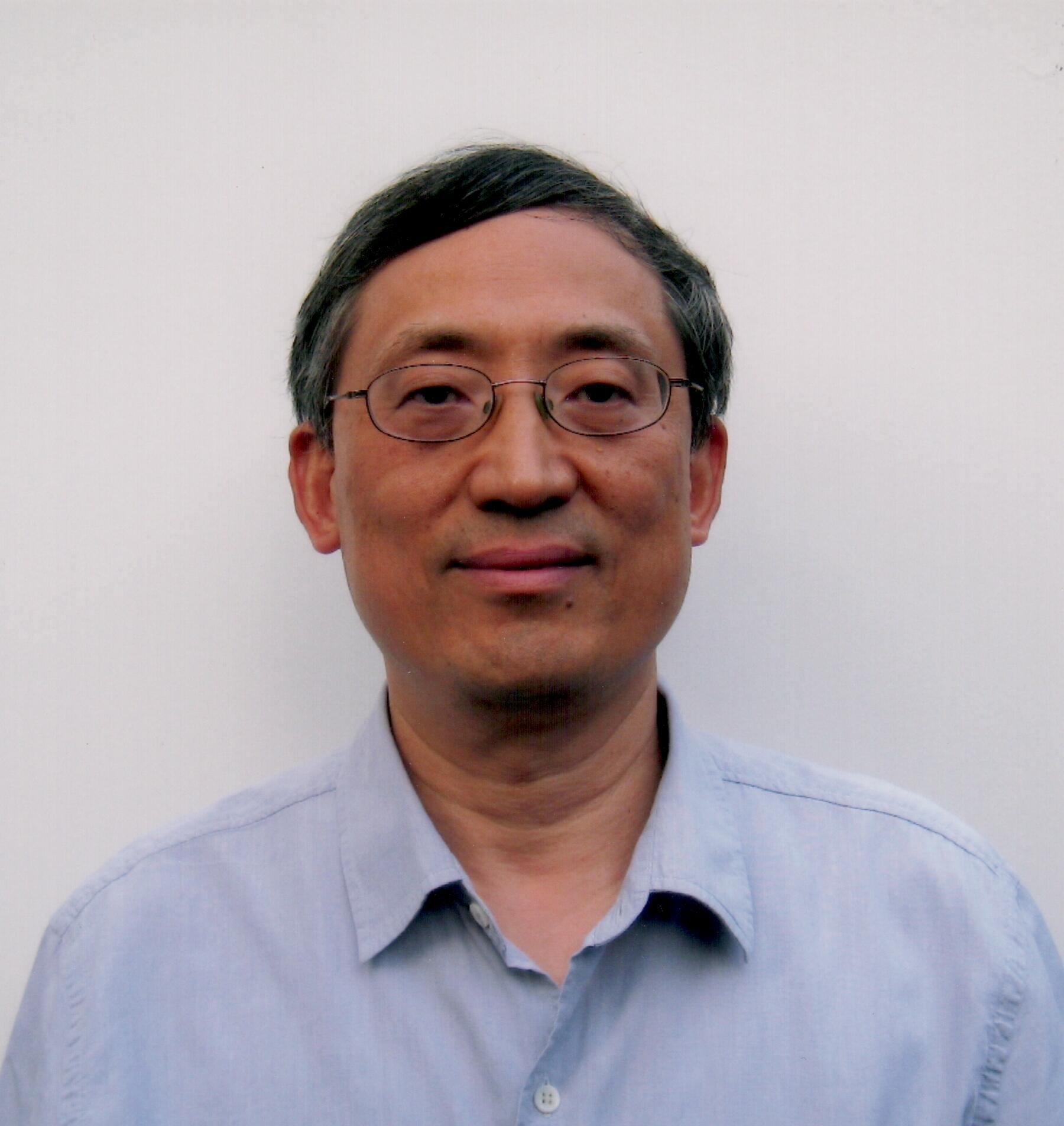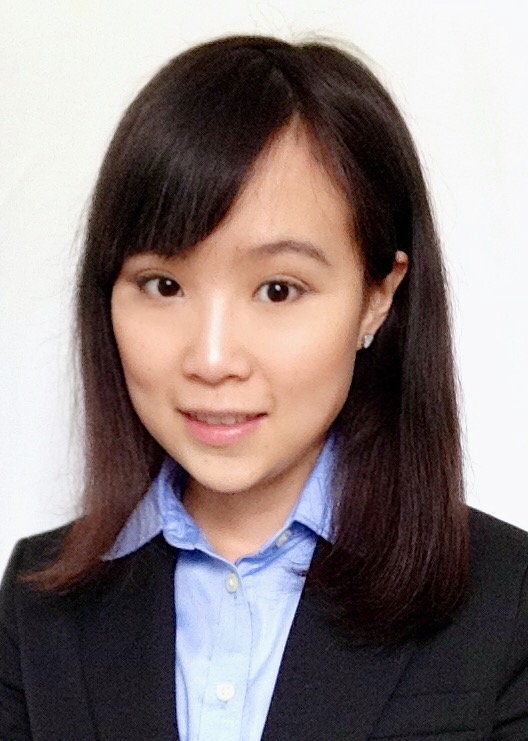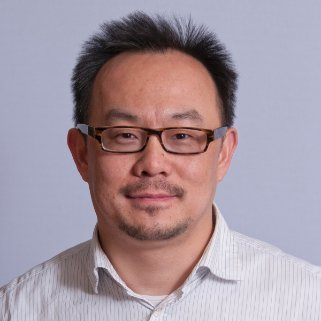# Technical Program (to be finalized)

The registration is open on August 13, 16:00 - 19:00, at DaoYuan Building Lobby.

The opening ceremony takes place on August 14, 8:15 - 8:30, at Room 103 Chengdao Building.

• August 14: David Tse and Yao Xie

• August 15: Lizhong Zheng and Dongxiao Zhu

• August 16: Yin Zhang and Andy Sun

# Plenary Talks (8:30–9:30)

## A Maximum Entropy Approach to Supervised LearningDavid Tse Stanford University

We develop a maximum entropy approach to supervised learning with general loss functions. While for some loss functions such as squared-error and log loss, the maximum entropy approach re-derives well-known regression models, for the 0-1 loss it results in a new linear classifier which we call the maximum entropy machine. In contrast to the empirical risk minimization framework, where computing the optimal linear classifier under the 0-1 loss is NP-hard, computing the maximum entropy machine is a convex optimization problem that can be solved efficiently.

## A Geometric Framework for Feature Selection of High Dimensional MultiModal DataLizhong Zheng Massachusetts Institute of Technology

In this talk, we present an overview of a new geometric framework for the general problems of selecting informative features from high dimensional data. The key message is that such problems need to be studied in the space of distributions of the data, for which, we develop a geometric structure that provides powerful insights. With this approach, we discuss a few different formulations of feature selection: as a universal inference problem with unknown statistical models, as generalized Renyi maximal correlation, or as decomposition of common information into ‘‘orthogonal modes”. We show that these different formulations share the same structure of solution, which is a SVD (Singular Value Decomposition) structure with strong geometric insights. This effort to connect different formulations helps to establish new operational meanings of information metrics in the context of feature selection and inference, as well as defining new semantic-aware information metrics. We demonstrate an algorithm designed based on this approach, which is flexible enough to handle any data type, particularly multi-modal data with different types, time-scales, and qualities. It offers provably the optimal inference performance, as well as the minimum sample complexity. We also show that the theoretic framework based on the geometric structure can be used to understand many existing feature selection algorithms, including PCA, CCA, Compressed Sensing, and Logistic regression.

## A matrix completion algorithm inspired by numerical linear algebraYin Zhang The Chinese University of Hong Kong, Shenzhen

The problem of minimizing the rank of a matrix arises in many applications, for example, in control and systems theory, model reduction, recovering shape and motion from image streams, data mining, pattern recognitions, and other machine learning tasks such as latent semantic indexing and low-dimensional embedding. The matrix completion problem, in its purest form, is to minimize the rank of a matrix given a subset of its entries. The main solution strategy for this problem has been based on nuclear-norm minimization which requires computing singular value decompositions – a task that is increasingly costly as matrix sizes and ranks increase. To improve the capacity of solving large-scale problems, we propose to solve a low-rank factorization model corresponding to a nonconvex optimization problem. This model naturally lends itself to an alternating minimization scheme corresponding to a nonlinear Gauss-Seidel algorithm. We use an idea from numerical linear algebra to construct a nonlinear successive over-relaxation (SOR) algorithm that only requires solving a single linear least squares problem per iteration. Extensive numerical experiments show that the algorithm can reliably solve a wide range of problems at a speed at least several times faster than some popular nuclear-norm minimization algorithms. In addition, convergence of this nonlinear SOR algorithm to a stationary point is analyzed.

# Tutorials (9:40–12:00; 13:30–17:30)

## Computational StatisticsYao Xie Georgia Institute of Technology

Computational statistic is an interface between statistics and computing. We will cover statistical models and computational methods, including Gaussian mixture model, Hidden Markov models (HMM), EM algorithm, model selection and cross validation, and sequential methods such as change-point detection.

## A survey of statistical learning techniques with applications to bioinformatics and health informaticsDongxiao Zhu Wayne State University

In this tutorial, I will first review an array of popular statistical learning techniques, such as Lasso, fused Lasso, group Lasso and elastic net, for learning from high dimension data through model regularization. I will then introduce three new extensions of these approaches to solve unique bioinformatics and health informatics problems, which includes (1) a Multinomial Sparse Overlapping Group Lasso to solve cancer classification problem using overlapping biological pathway information; (2) a Robust Feature Selection via Sparse l2,1-Norm in Finite Mixture of Regression to solve the problem of mixture distribution target in regression; and (3) an Elastic-Net type of approach to convex and sparse optimization to solve a supervised biclustering problem. Finally I will discuss a number of real-world applications in bioinformatics and health informatics.

## Introduction to Stochastic Optimization: from Basic Models to Advanced AlgorithmsAndy Sun Georgia Institute of Technology

Stochastic optimization is one of the cornerstones of modern analytical methods with wide applications in operations research, decision sciences, stochastic control, statistical learning, finance, and engineering. In this tutorial, we will introduce fundamental models, such as two-stage and multistage stochastic linear and integer programs, data-driven reformulation techniques, such as chance-constrained programming, and various solution algorithms including sampling based methods and decomposition algorithms for solving large-scale stochastic optimization. We will start from the basic models, connect theory with applications in statistical learning and engineering, and introduce the students to the most recent developments in the research frontier.